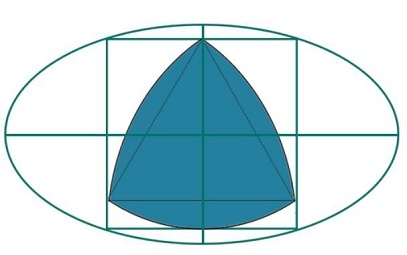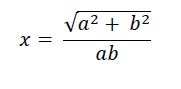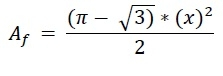# Biggest Reuleaux Triangle inscribed within a square which is inscribed within an ellipse?

Here we will see the area of biggest Reuleaux triangle inscribed within a square, that square is inscribed inside one ellipse. We know that the major axis length is 2a, and the minor axis length is 2b. The side of the square is ‘x’, and the height of the Reuleaux triangle is h.We know that the side of a square inscribed in an ellipse with major axis 2a and minor axis 2b is −The height of the Reuleaux triangle is same as a. So h = x. So the area of Reuleaux triangle is −.

## Example

#include <iostream>
#include <cmath>
using namespace std;
float areaReuleaux(float a, float b) { //a and b are half of major and minor axis of ellipse
if (a < 0 || b < 0) //either a or b is negative it is invalid
return -1;
float x = sqrt((a*a) + (b*b)) / (a*b);
float area = ((3.1415 - sqrt(3)) * (x) * (x))/2;
return area;
}
int main() {
float a = 5;
float b = 4;
cout << "Area of Reuleaux Triangle: " << areaReuleaux(a, b);
}

## Output

Area of Reuleaux Triangle: 0.0722343